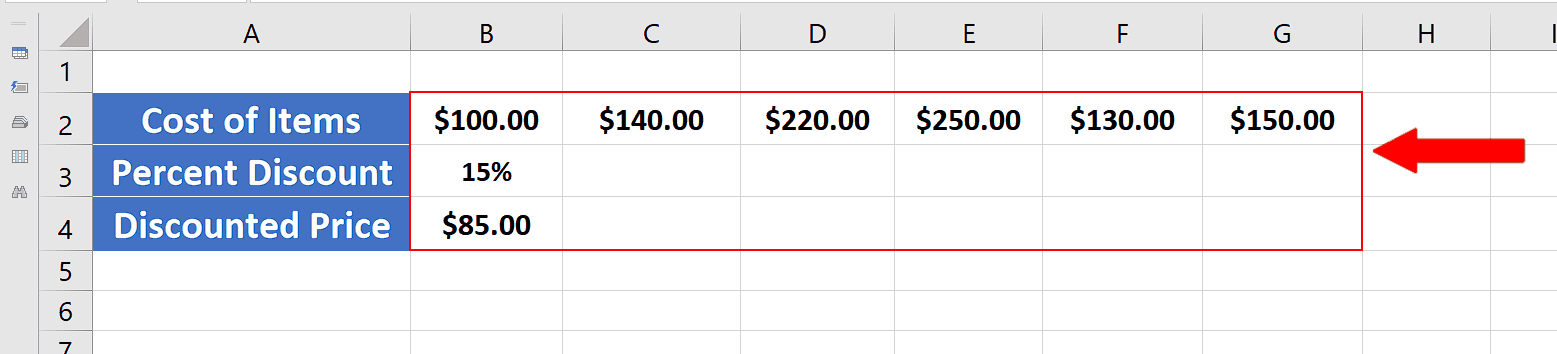# How to use CTRL+R in Excel

Let’s take a look at the following dataset in which we have the costs of different items and we wish to calculate the percent discount on each of them.

We have already implemented the formula to calculate the discounted price in the above dataset and calculated the first value. Now if we drag the formula across all cells starting from B4 to all the way to G4, we will not get the desired results because all the cells above these cells are empty. Let’s see how we can use CTRL+R to solve this problem.Excel is a very powerful tool to perform mathematical and statistical calculations on numeric data. It also provides a variety of tools and shortcut keys to perform functions quickly. In today’s tutorial we’ll learn how to use the shortcut key CTRL+R in Excel.

### Step 1 – Use CTRL+R to fill data across all cells– The data shows we have already calculated the discount on the first item and we wish to use the same percent discount for each item. In this scenario the shortcut key CTRL+R comes in very handy. We’ll select the first cell from B3 and extend our selection up to G3 and then press CTRL+R. This will fill all cells with 15% and the discounted price will be calculated automatically because the formula was already in place.